# MA.912.NSO.1.4Export Print
Apply previous understanding of operations with rational numbers to add, subtract, multiply and divide numerical radicals.

### Examples

Algebra 1 Example: The expression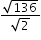is equivalent to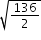which is equivalent to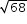which is equivalent to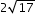.

### Clarifications

Clarification 1: Within the Algebra 1 course, expressions are limited to a single arithmetic operation involving two square roots or two cube roots.
General Information
Subject Area: Mathematics (B.E.S.T.)
Strand: Number Sense and Operations
Status: State Board Approved

## Benchmark Instructional Guide

### Terms from the K-12 Glossary

• Base
• Rational Number

### Vertical Alignment

Previous Benchmarks

Next Benchmarks

### Purpose and Instructional Strategies

In grade 8, students evaluated numerical expressions with square and cube roots. In Algebra I, students perform operations with numerical square or cube roots. In later courses, students will perform operations with algebraic expressions involving radicals.
• It is important to reinforce and activate the prior knowledge of simple calculations with radicals within this benchmark.
• Within this benchmark, it is not the expectation to rationalize the denominator. However, students should have experience and understanding how to rationalize the denominator. Students should understand when it may be helpful to rationalize, for example when estimating values.
• Instruction includes making the connection to properties of exponents with rational exponents.
• For example, when determining the value of the expression 4$\sqrt{3}$($\sqrt{3}$), a student could convert to exponential notation, which would result in 4(3$\frac{\text{1}}{\text{2}}$) × 3$\frac{\text{1}}{\text{2}}$. A student could then use properties of exponents to obtain 4(3$\frac{\text{1}}{\text{2}}$ + $\frac{\text{1}}{\text{2}}$) which is equivalent to 12.
• For example, when determining the value of the expression, a student could convert to exponential notation, which would result in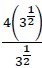. A student could then use properties of exponents to obtain 4(3$\frac{\text{1}}{\text{2}}$$\frac{\text{1}}{\text{2}}$) which is equivalent to 4.
• Instruction allows for students to write their answer in radical or exponential form.

### Common Misconceptions or Errors

• Students may not know how to do simple calculations with radicals, therefore, they may not take the square root of the perfect square factor; or they may suggest using a factor pair within a radical that does not contain a perfect square as a factor.
• Students may confuse radicands and coefficients and perform the operations on the wrong part of the expression.

### Strategies to Support Tiered Instruction

• Teacher provides opportunities to write out all of the factors, as sets, of the radicand. Once the factors have been identified, the students can use the set of factors with the highest perfect square or cube, as applicable.
• For example, given $\sqrt{200}$, the radicand is 200, the factor sets are {1 and 200}, {2 and 100}, {4 and 50}, etc., 100 is the highest perfect square; so, $\sqrt{200}$ = 10$\sqrt{2}$
• Teacher co-creates a list of common perfect squares and cubes that can be used as problems are performed.
• For example, a list of common perfect squares and cubes is shown.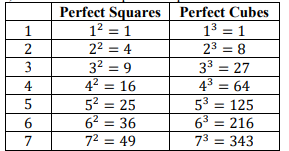• Teacher models identifying all parts of the radical expression and rules specific to the operation prior to calculating answers. To add or subtract radicals the radicand must be the same prior to adding the coefficients.
• For example, given 6$\sqrt{20}$ + 4$\sqrt{20}$, 6 and 4 are coefficients and 20 is the radicand for both terms. Therefore, 6$\sqrt{20}$ + 4$\sqrt{20}$ = 10$\sqrt{20}$
• For example, given 4$\sqrt{5}$ − 9$\sqrt{20}$, 4 and 9 are coefficients and 5 and 20 are the radicands. Therefore, the terms cannot be combined as is. But notice that if $\sqrt{20}$ is rewritten as 2$\sqrt{5}$, then 5 is the radicand for both terms. So, 4$\sqrt{5}$ − 9$\sqrt{20}$ which is equivalent to 4$\sqrt{5}$ − 18$\sqrt{5}$ which when subtracted results in −14$\sqrt{5}$
• Teacher provides a visual aid (e.g., laminated cue card) to distinguish the parts of a radical expressions.
• For example, in the expression $\sqrt{20}$, 3 is the index (or root) and 20 is the radicand.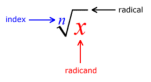• The velocity, $v$, measured in meters per second of an object can be measured in terms of its mass, $m$, measured in kilograms and Kinetic Energy, $E$$k$, measured in Joules. The equation below describes this relationship.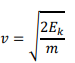• Part A. What is the exact velocity of an object if its mass measures 31 kilograms and its Kinetic Energy measures 310 Joules?
• Part B. Rearrange the formula to highlight mass as the quantity of interest.
• Part C. What is the mass of an object if the velocity measures 6.1 meters per second and its Kinetic Energy measures 31.4 Joules?

### Instructional Items

Instructional Item 1
• Determine the value of the expression $\sqrt{24}$ + $\sqrt{81}$. Write your answer as an exact quantity using only a single radical.
Instructional Item 2
• Determine the difference of the expression $\sqrt{24}$ − 2$\sqrt{54}$. Write your answer as an exact quantity using only a single radical.
Instructional Item 3
• Determine the value of the expression 4$\sqrt{10}$(−3$\sqrt{15}$). Write your answer as an exact quantity using only a single radical.
Instructional Item 4
• Determine the value of the expression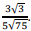*The strategies, tasks and items included in the B1G-M are examples and should not be considered comprehensive.

## Related Courses

This benchmark is part of these courses.
1200310: Algebra 1 (Specifically in versions: 2014 - 2015, 2015 - 2022, 2022 and beyond (current))
1200320: Algebra 1 Honors (Specifically in versions: 2014 - 2015, 2015 - 2022, 2022 and beyond (current))
1200380: Algebra 1-B (Specifically in versions: 2014 - 2015, 2015 - 2022, 2022 and beyond (current))
1200400: Foundational Skills in Mathematics 9-12 (Specifically in versions: 2014 - 2015, 2015 - 2022, 2022 and beyond (current))
7912090: Access Algebra 1B (Specifically in versions: 2014 - 2015, 2015 - 2018, 2018 - 2019, 2019 - 2022, 2022 and beyond (current))
1200315: Algebra 1 for Credit Recovery (Specifically in versions: 2014 - 2015, 2015 - 2022, 2022 and beyond (current))
1200385: Algebra 1-B for Credit Recovery (Specifically in versions: 2014 - 2015, 2015 - 2022, 2022 and beyond (current))
7912075: Access Algebra 1 (Specifically in versions: 2014 - 2015, 2015 - 2018, 2018 - 2019, 2019 - 2022, 2022 and beyond (current))

## Related Access Points

Alternate version of this benchmark for students with significant cognitive disabilities.
MA.912.NSO.1.AP.4: Apply previous understanding of operations with rational numbers to add and subtract numerical radicals that are in radical form.

## Related Resources

Vetted resources educators can use to teach the concepts and skills in this benchmark.